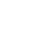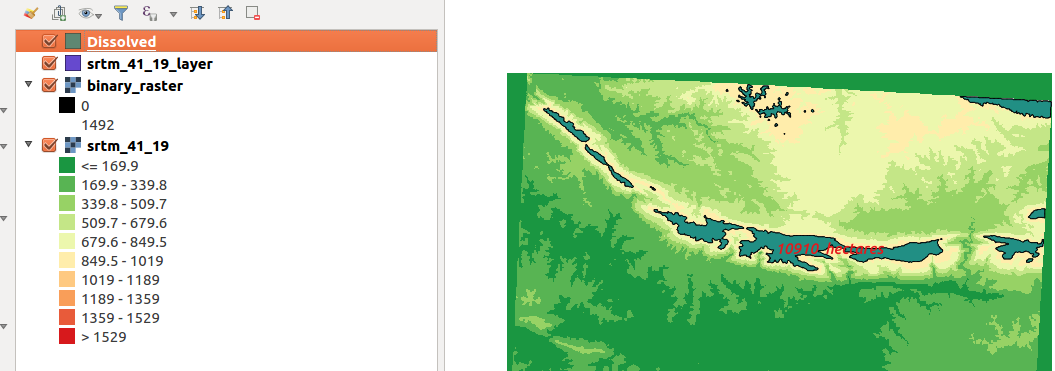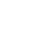### Vectorisation in Context

“Vectorization is the process of converting a raster into a vector.”

In this module, we will learn how to mask a raster layer and then convert the AOI(area of interest) into a vector. Vector geometry is easy to manipulate. Because of the ease of using vector geometry, we sometimes convert raster into a vector so that we can proceed with analysis.#### Silakan dicoba:

Tujuan To learn how to create mask raster in QGIS and doing raster statistics.

1 Load the raster layer specified.

2. Use the raster calculator to create a mask showing cells > 1000m = 1, and cells < 1000m = 0

3. Symbolize the raster into two discrete classes.

4. Assign a no data value to the dem_mask layer so that you only show where cell values are 1.

NB: How can you calculate the area where all the cell values are 0 or 1.

5. Convert the mask layer to vector.

6. Dissolve the vector layer and edit the result to add a column for calculating area.

7. Use the expression for calculating the area and convert the total area to hectares.

8. label the resultant polygon with the area

9. Repeat the procedure from step 4 - step 8. Change the no-data value to 1.

Nama Ekspektasi

Data

srtm/ srtm_41_19.tif

Expression

\$area

( "[email protected]" >= 1000 ) * "[email protected]"

No Data value

0Raster data contains cell values which represent a spatial phenomenon at the cell location. Raster operations performed on multiple input raster datasets generally output cell values that are the result of computations on a cell-by-cell basis.

Raster analysis is mainly grouped into three categories which are local operations, focal operations and zonal operations. Local operations allow manipulating of the vector values by doing arithmetic operations for example reclassification to produce a binary raster. Neighbourhood operations make use of the topological relationship of adjacency between cells in the input raster to create a new raster layer.

Because raster images are generally big with a large area coverage any operations (focal, zonal and local) take time to complete and they are also influenced by the resolution of the raster image. Converting the raster to vector provides an easy option where computations are very fast.### Periksa pemahamanmu:

1. When is it necessary to convert a raster to vector:
1. When the raster layer is a satellite image
2. When we have derived new information and such that further analysis require data to be combined with other vector sources.
3. It is not recommended to convert a raster to vector.
2. Why would you want to have two different map layouts using the same layer:
1. Each cell value in a raster corresponds to a specific polygon in a vector
2. Vectorisation can only be done on a raster that has been classified or a raster layer that is a mask.
3. Vectors generated from rasters are not normal vector data.
3. Can you calculate total area for raster cells greater than 100m without converting to vector:
1. True
2. False